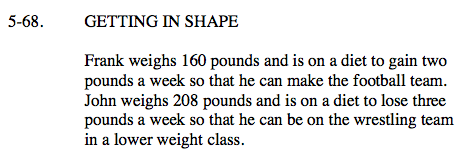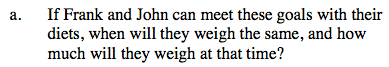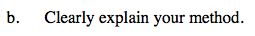### Home > CAAC > Chapter 5 > Lesson 5.2.1 > Problem5-68

5-68.Use the information in the problem to set up
y = mx + b equations for both Frank and John.

Set the equations equal to each other and solve for x.

Substitute x back into one of the original equations and solve for y. Check that x and y work in both equations.